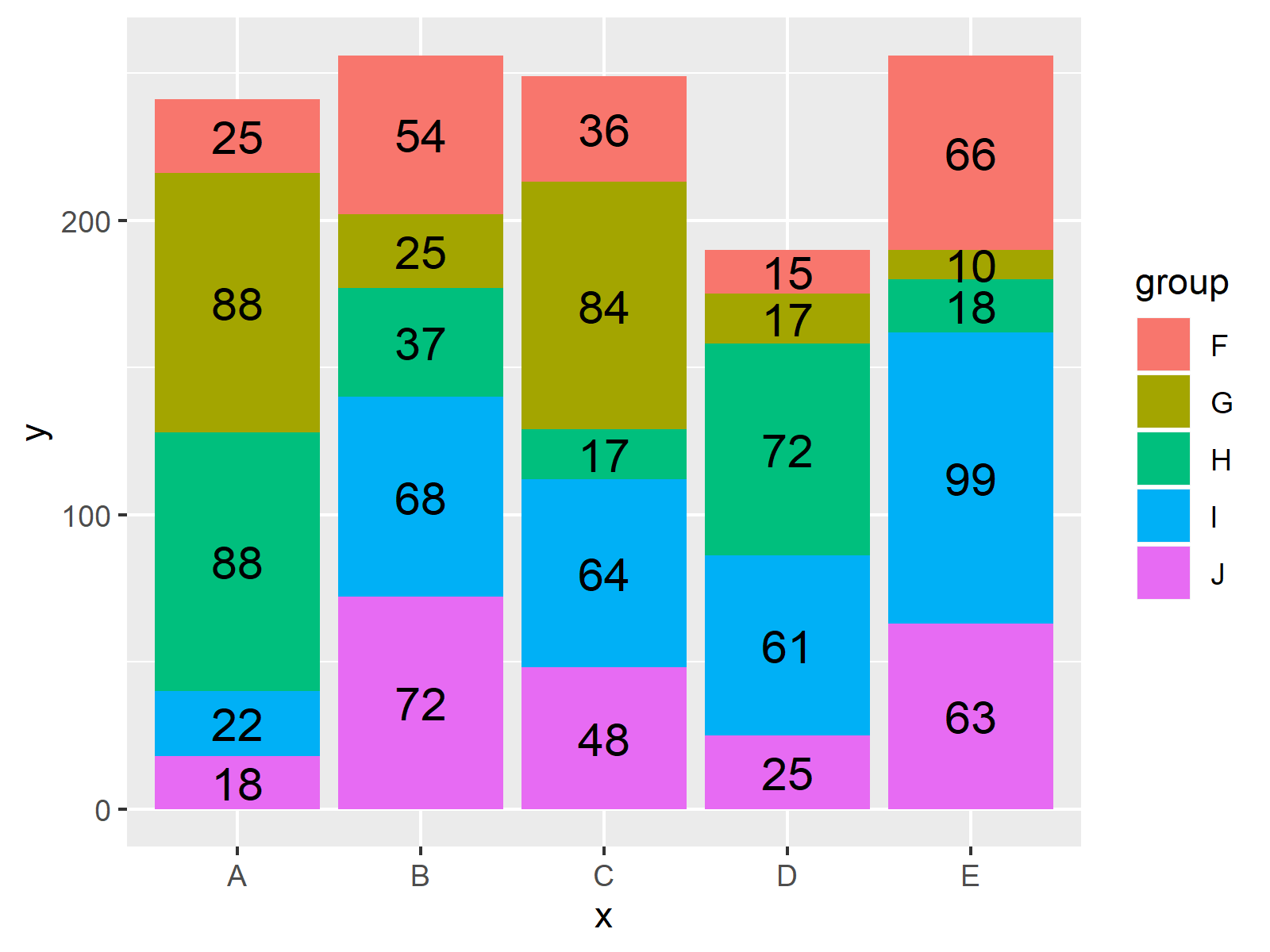# Plot Frequencies on Top of Stacked Bar Chart with ggplot2 in R (Example)

In this R programming tutorial you’ll learn how to show data values on top of each bar of a stacked ggplot2 bar chart.

The post contains one example for the plotting of data with ggplot2. To be more specific, the post consists of this content:

Sound good? Let’s jump right to the R code:

## Creating Exemplifying Data

First, we need to create some example data that we can plot in a bar chart:

```set.seed(85158)                                                    # Create example data
data <- data.frame(x = rep(LETTERS[1:5], each = 5),
y = round(runif(25, 10, 100)),
group = rep(LETTERS[6:10], time = 5))
#   x  y group
# 1 A 25     F
# 2 A 88     G
# 3 A 88     H
# 4 A 22     I
# 5 A 18     J
# 6 B 54     F```

As you can see based on the output of the RStudio console, our example data consists of three columns: x, y and a grouping column.

If we want to draw a plot of our data with the ggplot2 package, we also need to install and load the ggplot2 package:

```install.packages("ggplot2")                                        # Install and load ggplot2
library("ggplot2")```

Now, we can draw a ggplot2 stacked bar graph as follows:

```ggp <- ggplot(data, aes(x = x, y = y, fill = group, label = y)) +  # Create stacked bar chart
geom_bar(stat = "identity")
ggp                                                                # Draw stacked bar chart```Figure 1: Stacked Bar Chart Created with ggplot2 Package in R.

Figure 1 illustrates the output of the previous R code – A stacked bar chart with five groups and five stacked bars in each group.

Next, I’ll show how to add frequency values on top of each bar in this graph. So keep on reading!

## Example: Draw Stacked ggplot2 Bar Plot with Frequencies on Top

If we want to put values on the top of each bar of our bar chart, we have to use the geom_text function and the position_stack argument of the ggplot2 package. Have a look at the following R programming syntax:

```ggp +                                                              # Add values on top of bars
geom_text(size = 5, position = position_stack(vjust = 0.5))```Figure 2: ggplot2 Stacked Bar Chart with Frequencies on Top of Bars.

As you can see in Figure 2, we added the frequency numbers of each bar on top. Looks great!

## Video & Further Resources

Do you need more information on the R codes of this tutorial? Then you might watch the following video of my YouTube channel. I’m explaining the R programming codes of this tutorial in the video:

Please accept YouTube cookies to play this video. By accepting you will be accessing content from YouTube, a service provided by an external third party.If you accept this notice, your choice will be saved and the page will refresh.

In addition, you may want to read some of the other articles of this website. I have released several posts already:

To summarize: This tutorial showed how to plot a frequencies, proportion values or a percentage on the top of each bar of a ggplot2 bar graphic in the R programming language. In case you have additional comments or questions, please let me know in the comments section.

Subscribe to the Statistics Globe Newsletter

•Julio Souza
March 23, 2021 12:57 pm

is nice exemple.
Julio

•March 23, 2021 1:11 pm

Thanks a lot Julio, great to hear that you liked it!

Regards

Joachim

•walter
May 22, 2022 2:50 pm

Hey I have a specific question regarding stacked using ggplot,It goes as follow : here is the code

datt <- read.table(text = " Architektur Biologie Mathematik
Bayern 4377 7295 7014
Berlin 2929 2969 3449
Brandenburg 1148 982 825
Bremen 427 877 738
Hamburg 574 1513 506
Hessen 5155 4426 7617
Mecklenburg-Vorpommern 445 1468 537
Niedersachsen 3102 5353 5464
Nordrhein-Westfalen 9453 14356 18247
Rheinland-Pfalz 1916 2907 3235
Saarland 296 381 334
Sachsen 1182 1354 1521
Sachsen-Anhalt 758 543 349
Schleswig-Holstein 438 1239 1336
Thüringen 1796 921 445

library(reshape2)
#Zeile
datt\$row <- seq_len(nrow(datt))
#
ww<- melt(datt, id.vars = "row")
ww
library(ggplot2)

ggplot(ww , aes(x = variable, y = value, fill = row) ) +
geom_bar( stat = "identity") +
xlab("\nStudienfach WS2019/20") + ylab("Absolute Häufigkeit\n") +

ggtitle("Drei Studiengängen in den verschiedenen Bundesländern WS2019/22")+
theme(plot.title = element_text(hjust=0.5))
+ geom_text(size=3,position = position_stack(vjust = 0.5))

These are my questions:
with the above code 1. How can I change the colours of the stacked to more distinguished colours.
2. Adding the values on the stacked
3.modifying my legend and the name of the legend.
I really try everything so far and also look at almost all of your videos on stacked barplot,but having been able to do it.Something is lacking me.I also try it with a barplot just like you did in one of your video with as.matrix.I will post it as a different question.
I will really appreciate your help.

•May 23, 2022 6:49 am

Hey Walter,

Could you clarify what information you would like to show…
– on the x-axis
– on the y-axis
– within the different stacked bars

Regards,
Joachim